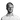# 13 JavaScript One-Liners That’ll Make You Look Like a Pro

JavaScript

24 January, 2021 • ☕️ 3 min read

JavaScript can do a lot of amazing things!

From complex frameworks to handling API’s, there is just SO much to learn.

But, it also enables you to do some awesome stuff using just one single line.

Check out these 13 JavaScript One-Liners That’ll Make You Look Like a Pro!

## 1. Get a random boolean (true/false)

This function will return a boolean (true or false) using the `Math.random()` method. `Math.random` will create a random number between 0 and 1, after which we check if it is higher or lower than 0.5. That means it’s a 50%/50% chance to get either true or false.``````const randomBoolean = () => Math.random() >= 0.5;

console.log(randomBoolean());
// Result: a 50/50 change on returning true of false``````

## 2. Check if the provided day is a weekday

Using this method, you’ll be able to check if the date that you provide in the function is either a weekday or weekend day.``````const isWeekday = (date) => date.getDay() % 6 !== 0;

console.log(isWeekday(new Date(2021, 0, 11)));
// Result: true (Monday)

console.log(isWeekday(new Date(2021, 0, 10)));
// Result: false (Sunday)``````

## 3. Reverse a string

There are a couple different ways to reverse a string. This is one of the most simple ones using the `split()`, `reverse()`, and `join()` methods.``````const reverse = str => str.split('').reverse().join('');

reverse('hello world');
// Result: 'dlrow olleh'``````

## 4. Check if the current tab is in view / focus

We can check if the current tab is in view / focus by using the `document.hidden` property.``````const isBrowserTabInView = () => document.hidden;

isBrowserTabInView();
// Result: returns true or false depending on if tab is in view / focus``````

## 5. Check if a number is even or odd

A super simple task that can be solved by using the modulo operator (`%`). If you’re not too familiar with it, here’s a nice visual explanation on Stack Overflow.``````const isEven = num => num % 2 === 0;

console.log(isEven(2));
// Result: true

console.log(isEven(3));
// Result: false``````

## 6. Get the time from a date

By using the `.toTimeString()` method and slicing the string at the correct place, we can get the time from a date that we provide, or get the current time.``````const timeFromDate = date => date.toTimeString().slice(0, 8);

console.log(timeFromDate(new Date(2021, 0, 10, 17, 30, 0)));
// Result: "17:30:00"

console.log(timeFromDate(new Date()));
// Result: will log the current time``````

## 7. Truncate a number to a fixed decimal point

Using the `Math.pow()` method, we can truncate a number to a certain decimal point that we provide in the function.``````const toFixed = (n, fixed) => ~~(Math.pow(10, fixed) * n) / Math.pow(10, fixed);

// Examples
toFixed(25.198726354, 1);       // 25.1
toFixed(25.198726354, 2);       // 25.19
toFixed(25.198726354, 3);       // 25.198
toFixed(25.198726354, 4);       // 25.1987
toFixed(25.198726354, 5);       // 25.19872
toFixed(25.198726354, 6);       // 25.198726``````

## 8. Check if an element is currently in focus

We can check if an element is currently in focus using the `document.activeElement` property.``````const elementIsInFocus = (el) => (el === document.activeElement);

elementIsInFocus(anyElement)
// Result: will return true if in focus, false if not in focus``````

## 9. Check if the current user has touch events supported``````const touchSupported = () => {
('ontouchstart' in window || window.DocumentTouch && document instanceof window.DocumentTouch);
}

console.log(touchSupported());
// Result: will return true if touch events are supported, false if not``````

## 10. Check if the current user is on an Apple device

We can use `navigator.platform` to check if the current user is on an Apple device.``````const isAppleDevice = /Mac|iPod|iPhone|iPad/.test(navigator.platform);

console.log(isAppleDevice);
// Result: will return true if user is on an Apple device``````

## 11. Scroll to top of the page

The `window.scrollTo()` method will take an x- and y-coordinate to scroll to. If we set these to zero and zero, we’ll scroll to the top of the page.

Note: the`.scrollTo()`method isn’t supported on Internet Explorer.``````const goToTop = () => window.scrollTo(0, 0);

goToTop();
// Result: will scroll the browser to the top of the page``````

## 12. Get average value of arguments

We can use the reduce method to get the average value of the arguments that we provide in this function.``````const average = (...args) => args.reduce((a, b) => a + b) / args.length;

average(1, 2, 3, 4);
// Result: 2.5``````

## 13. Convert Fahrenheit / Celsius

The final one is a 2-in-1!

Dealing with temperatures can be confusing at times. These 2 functions will help you convert Fahrenheit to Celsius and the other way around.``````const celsiusToFahrenheit = (celsius) => celsius * 9/5 + 32;
const fahrenheitToCelsius = (fahrenheit) => (fahrenheit - 32) * 5/9;

// Examples
celsiusToFahrenheit(15);    // 59
celsiusToFahrenheit(0);     // 32
celsiusToFahrenheit(-20);   // -4

fahrenheitToCelsius(59);    // 15
fahrenheitToCelsius(32);    // 0``````

Thanks for reading! I hope you learned something new today.

Want to improve your JS skills even more? Check out my other article “The 7 JS Array Methods you will need in 2021” or “What’s the difference between Event Handlers & addEventListener in JS?” to get even better at JS.

Have a nice day! 😄